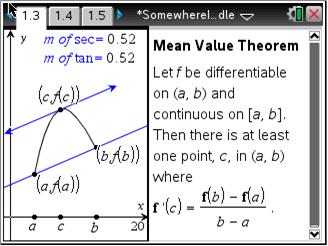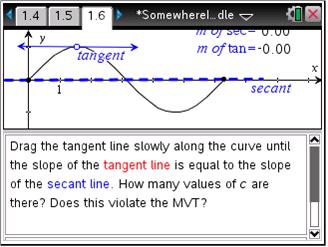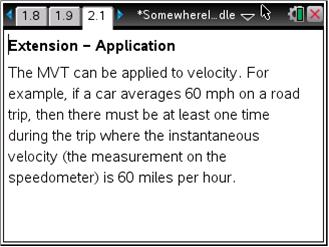# Activities

••• ##### Subject Area

• Math: Calculus: Applications of the Derivative
• Math: Calculus: Derivatives

• ##### Author9-12

15 Minutes

• ##### Device
•TI-Nspire™ CX/CX II
•TI-Nspire™ CX CAS/CX II CAS
• TI-Nspire™
• TI-Nspire™ CAS
• ##### Software

TI-Nspire™
TI-Nspire™ CAS

3.2

## Somewhere in the Middle

#### Activity Overview

In this activity, students will explore the Mean Value Theorem. Students will find out when the tangent line is parallel to the secant line passing through the endpoints of an interval to help them find the values of c guaranteed to exist by the MVT. Students will also test functions where the hypotheses of the MVT are not met.

#### Key Steps

•Students are introduced to the Mean Value Theorem.

•Students use the tangent and secant lines to find the value of c for several different functions, some of which have more than one value for c, or have no value for c.

•In an extension, students apply the Mean Value Theorem to prove that two horses had the same velocity during a race.